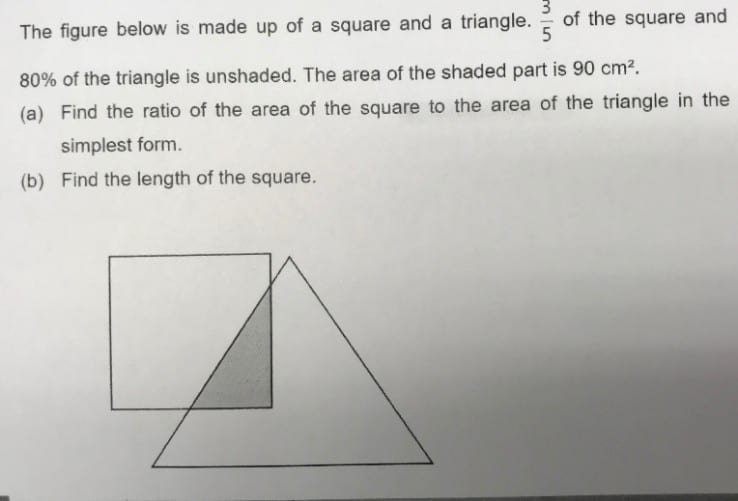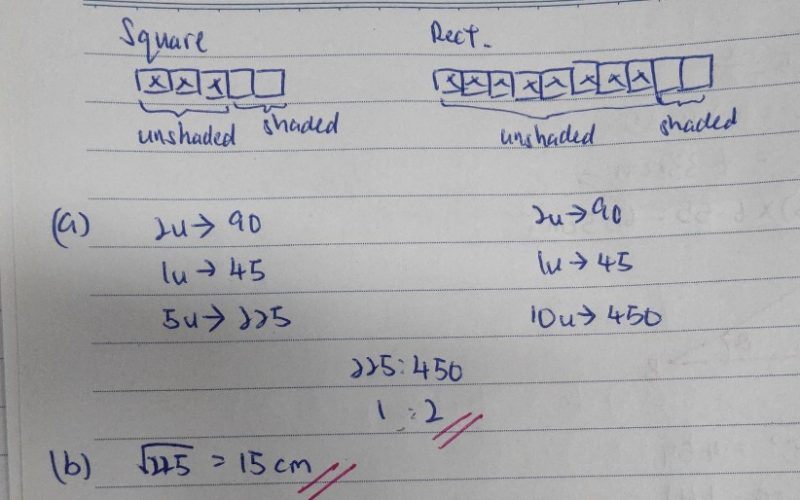QuestionHi, I need some help. Have been struggling to assist my kid with this problem. Thanks in advance.

Make Numerator the same

2u = 90

1u = 45

Area of Square is 5u = 225

Area of Triangle is 10u = 450

a) Ratio of area of square to area of triangle

225:450

1:2 (Ans)

b) Area of Square = 225 cm^2 = 15 cm x 15 cm

Length of Square = 15 cm (Ans)

0 Replies 1 Like ✔Accepted Answer

(a)
For square,
3 : 2 : 5

90 : 225

For triangle,
8 : 2 : 10

90 : 450

area of the square : area of the triangle
225 : 450
1 : 2

(b)
225 = 15 x 15

Ans : (a) 1 : 2 ; (b) 15 cm.

0 Replies 1 Like